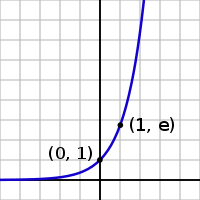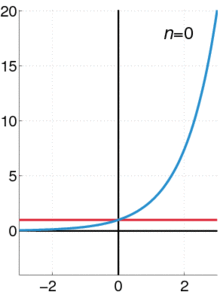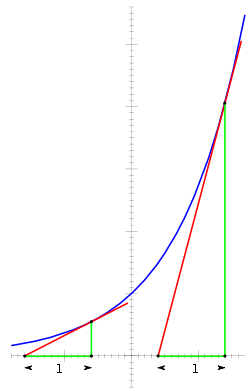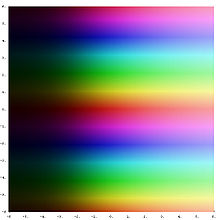# Exponential function

Exponential functionThe natural exponential function y = ex

In mathematics, the exponential function is the function ex, where e is the number (approximately 2.718281828) such that the function ex is its own derivative. The exponential function is used to model a relationship in which a constant change in the independent variable gives the same proportional change (i.e. percentage increase or decrease) in the dependent variable. The function is often written as exp(x), especially when it is impractical to write the independent variable as a superscript.

 Exponential Function Representation$e^x \,$ Inverse$\ln x \,$ Derivative$e^x \,$ Indefinite Integral$e^x + C \,$

The graph of y = ex is upward-sloping, and increases faster as x increases. The graph always lies above the x-axis but can get arbitrarily close to it for negative x; thus, the x-axis is a horizontal asymptote. The slope of the tangent to the graph at each point is equal to its y coordinate at that point. The inverse function is the natural logarithm ln(x); because of this, some old texts[which?] refer to the exponential function as the antilogarithm.

Sometimes the term exponential function is used more generally for functions of the form cbx, where the base b is any positive real number, not necessarily e. See exponential growth for this usage.

In general, the variable x can be any real or complex number, or even an entirely different kind of mathematical object; see the formal definition below.

 Part of a series of articles on The mathematical constant eNatural logarithm · Exponential function Applications in: compound interest · Euler's identity & Euler's formula  · half-lives & exponential growth/decay People John Napier  · Leonhard Euler

## Overview

The exponential function arises whenever a quantity grows or decays at a rate proportional to its current value. One such situation is continuously compounded interest, and in fact it was this that led Jacob Bernoulli in 1683 to the number$\lim_{n \to \infty} \left(1+\frac{1}{n}\right)^n,$

now known as e. Later, in 1697, Johann Bernoulli studied the calculus of the exponential function.

If a principal amount of 1 earns interest at an annual rate of x compounded monthly, then the interest earned each month is x/12 times the current value, so each month the total value is multiplied by (1+x/12), and the value at the end of the year is (1+x/12)12. If instead interest is compounded daily, this becomes (1+x/365)365. Letting the number of time intervals per year grow without bound leads to the limit definition of the exponential function,$\exp(x) = \lim_{n\to\infty}\left(1+\frac{x}{n}\right)^{n},$

first given by Euler. This is one of a number of characterizations of the exponential function; others involve series or differential equations.

From any of these definitions it can be shown that the exponential function obeys the basic exponentiation identity,$\exp(x+y) = \exp(x) \cdot \exp(y),$

which is why it can be written as ex.

The derivative (rate of change) of the exponential function is the exponential function itself. More generally, a function with a rate of change proportional to the function itself (rather than equal to it) is expressible in terms of the exponential function. This function property leads to exponential growth and exponential decay.

The exponential function extends to an entire function on the complex plane. Euler's formula relates its values at purely imaginary arguments to trigonometric functions. The exponential function also has analogues for which the argument is a matrix, or even an element of a Banach algebra or a Lie algebra.

## Formal definitionThe exponential function (in blue), and the sum of the first n + 1 terms of the power series on the left (in red).

The exponential function ex can be characterized in a variety of equivalent ways. In particular it may be defined by the following power series:$e^x = \sum_{n = 0}^{\infty} {x^n \over n!} = 1 + x + {x^2 \over 2!} + {x^3 \over 3!} + {x^4 \over 4!} + \cdots.$

Using an alternate definition for the exponential function leads to the same result when expanded as a Taylor series.

Less commonly, ex is defined as the solution y to the equation$x = \int_1^y {dt \over t}.$

It is also the following limit:$e^x = \lim_{n \rightarrow \infty} \left(1 + \frac{x}{n}\right)^n.$

## Derivatives and differential equationsThe derivative of the exponential function is equal to the value of the function. From any point P on the curve (blue), if a tangent line (red), and a vertical line (green) are drawn as shown, then the triangle these lines make with the x-axis will have a base (green) of length 1. So the slope of the tangent line (the derivative) at that point P is equal to the height of the triangle (the value of the function).

The importance of the exponential function in mathematics and the sciences stems mainly from properties of its derivative. In particular,$\,{d \over dx} e^x = e^x.$

That is, ex is its own derivative and hence is a simple example of a Pfaffian function. Functions of the form cex for constant c are the only functions with that property (by the Picard–Lindelöf theorem) Other ways of saying the same thing include:

• The slope of the graph at any point is the height of the function at that point.
• The rate of increase of the function at x is equal to the value of the function at x.
• The function solves the differential equation y ′ = y.
• exp is a fixed point of derivative as a functional.

If a variable's growth or decay rate is proportional to its size—as is the case in unlimited population growth (see Malthusian catastrophe), continuously compounded interest, or radioactive decay—then the variable can be written as a constant times an exponential function of time. Explicitly for any real constant k, a function fRR satisfies f′ = kf if and only if f(x) = cekx for some constant c.

Furthermore for any differentiable function f(x), we find, by the chain rule:${d \over dx} e^{f(x)} = f'(x)e^{f(x)}.$

## Continued fractions for ex

A continued fraction for ex can be obtained via an identity of Euler:$\, \ e^x=1+\cfrac{x}{1-\cfrac{x}{x+2-\cfrac{2x}{x+3-\cfrac{3x}{x+4-\cfrac{4x}{x+5-\cfrac{5x}{x+6-\ddots}}}}}}$

The following generalized continued fraction for e2x/y converges more quickly:[citation needed]$e^{2x/y} = 1+\cfrac{2x}{y-x+\cfrac{x^2}{3y+\cfrac{x^2}{5y+\cfrac{x^2}{7y+\cfrac{x^2}{9y+\cfrac{x^2}{11y+\cfrac{x^2}{13y+\ddots\,}}}}}}}$

with a special case for x = y = 1:$e^2 = 7+\cfrac{2}{5+\cfrac{1}{7+\cfrac{1}{9+\cfrac{1}{11+\cfrac{1}{13+\ddots.}}}}}$

## Complex planeExponential function on the complex plane. The transition from dark to light colors shows that the magnitude of the exponential function is increasing to the right. The periodic horizontal bands indicate that the exponential function is periodic in the imaginary part of its argument.

As in the real case, the exponential function can be defined on the complex plane in several equivalent forms. Some of these definitions mirror the formulas for the real-valued exponential function. Specifically, one can still use the power series definition, where the real value is replaced by a complex one:$e^z = \sum_{n = 0}^\infty\frac{z^n}{n!}$

Using this definition, it is easy to show why$\textstyle {d \over dz} e^z = e^z$ holds in the complex plane.

Another definition extends the real exponential function. First, we state the desired property ex + iy = exeiy. For ex we use the real exponential function. We then use Euler's formula to define the latter factor: eiy = cos(y) + isin(y). Thus the real definition cannot be ignored.

When considered as a function defined on the complex plane, the exponential function retains the important properties

•$e^{z + w} = e^z e^w\,$
•$e^0 = 1\,$
•$e^z \ne 0$
•${d \over dz} e^z = e^z$

for all z and w.

The exponential function is an entire function as it is holomorphic over the whole complex plane. It takes on every complex number as value, except the value 0. This is an example of Picard's little theorem that any non-constant entire function takes on every complex number as value, except possibly one.

The exponential function is periodic with imaginary period i and can be written as$e^{a + bi} = e^a (\cos b + i \sin b)\,$

where a and b are real values (see also Euler's formula). This formula connects the exponential function with the trigonometric functions and to the hyperbolic functions. Thus all elementary functions except polynomials result from the exponential function in one way or another.

Extending the natural logarithm to complex arguments yields a multi-valued function, ln(z). We can then define a more general exponentiation:$z^w = e^{w \ln z}\,$

for all complex numbers z and w. This is also a multi-valued function. The above stated exponential laws remain true if interpreted properly as statements about multi-valued functions. By the way, the rule about multiplying exponents for positive real numbers does not work in multi-valued context:$(e^z)^w \ne e^\left(z w\right)$

See failure of power and logarithm identities for more about problems with combining powers.

The exponential function maps any line in the complex plane to a logarithmic spiral in the complex plane with the center at the origin. Two special cases might be noted: when the original line is parallel to the real axis, the resulting spiral never closes in on itself; when the original line is parallel to the imaginary axis, the resulting spiral is a circle of some radius.

### Computation of ab where both a and b are complex

Complex exponentiation ab can be defined by converting a to polar coordinates and using the identity (eln(a))b = ab:$\,a^b = (re^{{\theta}i})^b = (e^{\ln(r) + {\theta}i})^b = e^{(\ln(r) + {\theta}i)b}.$

However, when b is not an integer, this function is multivalued, because θ is not unique (see failure of power and logarithm identities).

## Matrices and Banach algebras

The power series definition of the exponential function makes sense for square matrices (for which the function is called the matrix exponential) and more generally in any Banach algebra B. In this setting, e0 = 1, and ex is invertible with inverse ex for any x in B. If xy =yx, then ex+y = exey, but this identity can fail for noncommuting x and y.

Some alternative definitions lead to the same function. For instance, ex can be defined as$\textstyle \lim_{n \to \infty} \left(1+\frac{x}{n} \right)^n$. Or ex can be defined as f(1), where fRB is the solution to the differential equation f′(t) = xf(t) with initial condition f(0) = 1.

## On Lie algebras

Given a Lie group G and its associated Lie algebra$\mathfrak{g}$, the exponential map is a map$\mathfrak{g}\to G$ satisfying similar properties. In fact, since R is the Lie algebra of the Lie group of all positive real numbers under multiplication, the ordinary exponential function for real arguments is a special case of the Lie algebra situation. Similarly, since the Lie group GL(n,R) of invertible n × n matrices has as Lie algebra M(n,R), the space of all n × n matrices, the exponential function for square matrices is a special case of the Lie algebra exponential map.

The identity exp(x+y) = exp(x)exp(y) can fail for Lie algebra elements x and y that do not commute; the Baker–Campbell–Hausdorff formula supplies the necessary correction terms.

## Double exponential function

The term double exponential function can have two meanings:

• a function with two exponential terms, with different exponents
• a function f(x) = aax; this grows even faster than an exponential function; for example, if a = 10: f(−1) = 1.26, f(0) = 10, f(1) = 1010, f(2) = 10100 = googol, ..., f(100) = googolplex.

Factorials grow faster than exponential functions, but slower than double-exponential functions. Fermat numbers, generated by$\,F(m) = 2^{2^m} + 1$ and double Mersenne numbers generated by$\,MM(p) = 2^{(2^p-1)}-1$ are examples of double exponential functions.

## Similar properties of e and the function ez

The function ez is not in C(z) (i.e., is not the quotient of two polynomials with complex coefficients).

For n distinct complex numbers {a1,..., an}, the set {ea1z,..., eanz} is linearly independent over C(z).

The function ez is transcendental over C(z).

Wikimedia Foundation. 2010.

### Look at other dictionaries:

• exponential function — Math. 1. the function y = ex. 2. any function of the form y = bax, where a and b are positive constants. 3. any function in which a variable appears as an exponent and may also appear as a base, as y = x2x. [1890 95] * * * In mathematics, a… …   Universalium

• exponential function — eksponentinė funkcija statusas T sritis fizika atitikmenys: angl. exponential function vok. exponentielle Funktion, f rus. экспоненциальная функция, f pranc. fonction exponentielle, f …   Fizikos terminų žodynas

• exponential function — noun a function in which an independent variable appears as an exponent (Freq. 1) • Syn: ↑exponential • Derivationally related forms: ↑exponential (for: ↑exponential) • Hypernyms: ↑ …   Useful english dictionary

• exponential function — noun Date: 1879 a mathematical function in which an independent variable appears in one of the exponents called also exponential …   New Collegiate Dictionary

• exponential function — noun Mathematics a function whose value is a constant raised to the power of the argument, especially the function where the constant is e …   English new terms dictionary

• exponential function — noun Any function in which an independent variable is in the form of an exponent; they are the inverse functions of logarithms …   Wiktionary

• Stretched exponential function — Figure 1. Illustration of a stretched exponential fit (with β=0.52) to an empirical master curve. For comparison, a least squares single and a double exponential fit are also shown. The data are rotational anisotropy of anthracene in… …   Wikipedia

• Double exponential function — A double exponential function (red curve) compared to a single exponential function (blue curve). A double exponential function is a constant raised to the power of an exponential function. The general formula is , which grows much more quickly… …   Wikipedia

• Ultra exponential function — Articleissues OR=January 2008 other=This article uses nonstandard notations, which are confusing and superfluous.In mathematics the ultra exponential function is a special case of the iterated exponential function more commonly known as tetration …   Wikipedia

• p-adic exponential function — In mathematics, particularly p adic analysis, the p adic exponential function is a p adic analogue of the usual exponential function on the complex numbers. As in the complex case, it has an inverse function named the p adic logarithm. Contents 1 …   Wikipedia